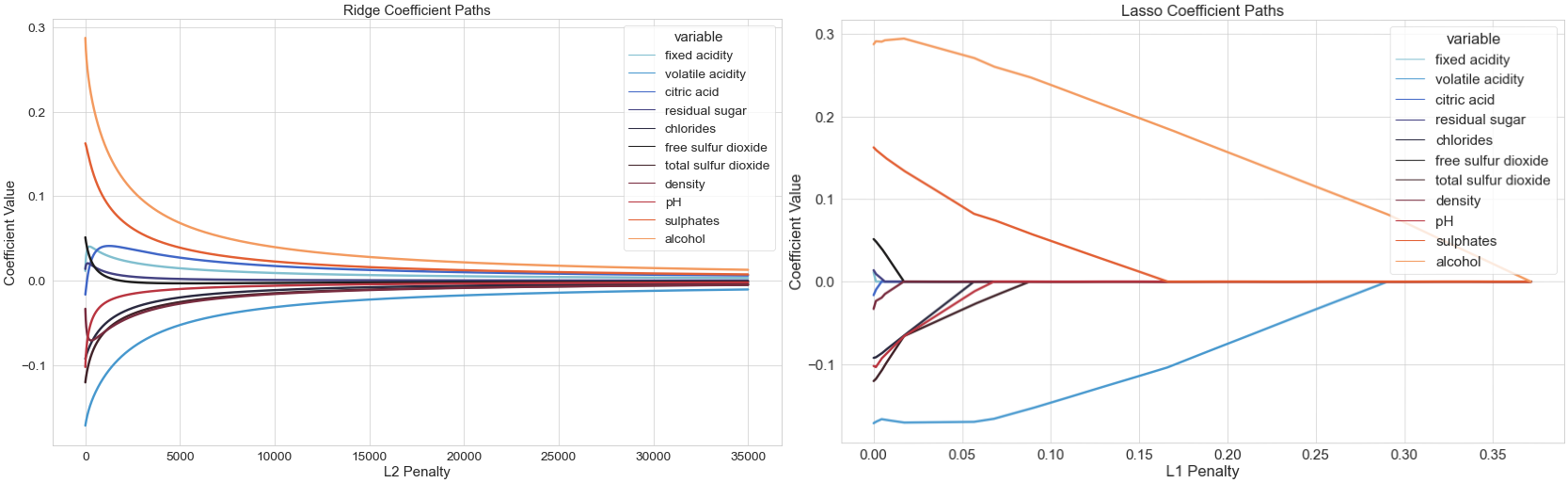# Publications

A collection of my published articles

### Article - Regularized Linear Regression Models: Implementing Pathwise Coordinate Descent For The Lasso and The Elastic Net In Python Using NumPy

Explanations for Solving Some of the Most Popular Supervised Learning Algorithms

January 2021### Article - Regularized Linear Regression Models: Using Ridge Regression to Overcome Drawbacks of Ordinary Least Squares (OLS)

Weaknesses of OLS, Optimization to Obtain the Ridge Model Estimator, and an Implementation in Python Using Numpy

January 2021### Article - Regularized Linear Regression Models: Basics of Linear Regression Modeling and Ordinary Least Squares (OLS)

Context of Linear Regression, Optimization to Obtain the OLS Model Estimator, and an Implementation in Python Using Numpy

January 2021Nifty tech tag lists from Wouter Beeftink